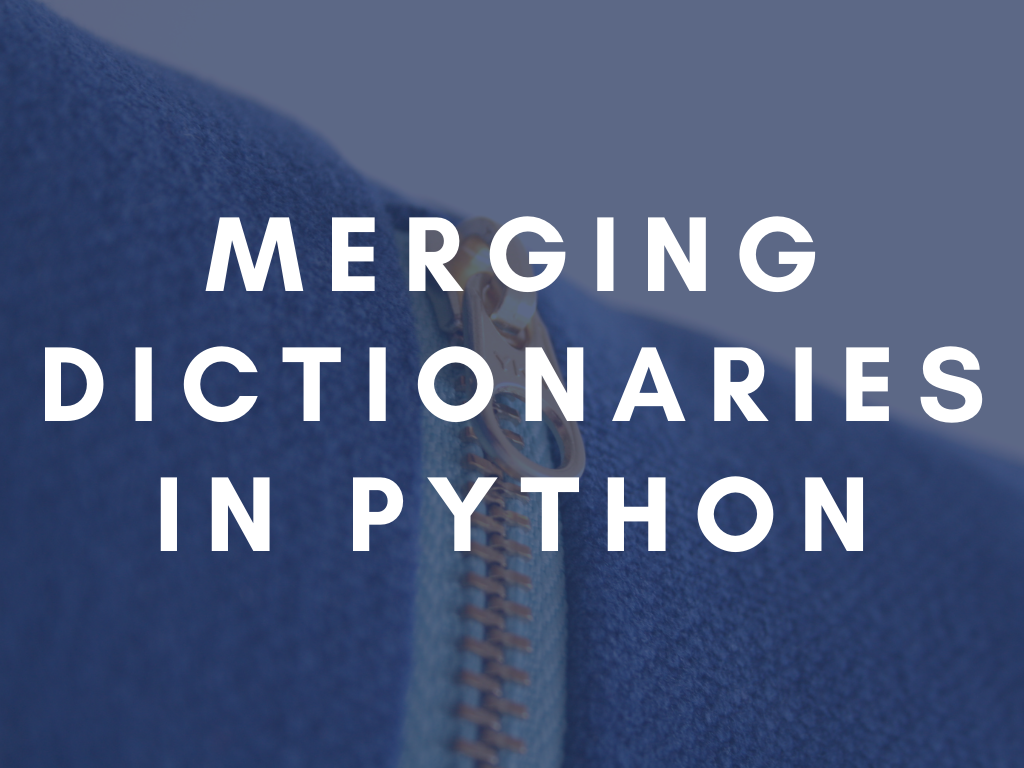# How To Merge Dictionaries In Python?Let’s say you have two (or more) dictionaries in Python and you want to unite them into a single dictionary containing the keys and values from both of the original dictionaries. How would you do that?

In Python 3.9 and above, you can use the merge operator: “merged = dict1 | dict2”. Starting from Python 3.5 you can unpack the two dictionaries and create a new one: “merged = {**dict1, **dict2}“. In older Python versions you would need to use a loop, or “dict.update()“.

Let’s look into these methods one-by-one with some examples

# Using a Loop

This is the naive approach, probably the least elegant of the solutions outlined here, but it works for every version of Python. We can just use a loop to iterate through all the keys and add the to the result dictionary one-by-one.

With two dictionaries:

``````dict1 = {'a': 1, 'b': 2}
dict2 = {'b': 3, 'c': 4}
merged = dict()
for key, value in dict1.items():
merged[key] = value
for key, value in dict2.items():
merged[key] = value``````

For multiple dictionaries you can just add an extra loop for each one.

``````dict1 = {'a': 1, 'b': 2}
dict2 = {'b': 3, 'c': 4}
dict3 = {'d': 5, 'e': 6}
merged = dict()
for key, value in dict1.items():
merged[key] = value
for key, value in dict2.items():
merged[key] = value
for key, value in dict3.items():
merged[key] = value``````

If you want to update one of the dictionaries in place, you can modify the code to do so:

``````dict1 = {'a': 1, 'b': 2}
dict2 = {'b': 3, 'c': 4}
for key, value in dict2.items():
dict1[key] = value``````

# Using dict.update

A more elegant way to achieve the same thing is to use `dict.update`. This method takes a dictionary and adds all its values to the dictionary it was called on. This method also works no matter what version of Python you use.

``````dict1 = {'a': 1, 'b': 2}
dict2 = {'b': 3, 'c': 4}
dict1.update(dict2)``````

Of course, it overwrites the contents of the original dictionary. If you want it to be unchanged you can create a copy and work on that:

``````dict1 = {'a': 1, 'b': 2}
dict2 = {'b': 3, 'c': 4}
merged = dict1.copy()
merged.update(dict2)``````

To merge multiple dictionaries, simply call `update` multiple times and pass the dicts one-by-one:

``````dict1 = {'a': 1, 'b': 2}
dict2 = {'b': 3, 'c': 4}
dict3 = {'d': 5, 'e': 6}
merged = dict1.copy()
merged.update(dict2)
merged.update(dict3)``````

# Unpacking the Dictionaries with the ** Operator

This is a much nicer - and more pythonic way to do things, however it will not work on Python versions older than Python 3.5.

``````dict1 = {'a': 1, 'b': 2}
dict2 = {'b': 3, 'c': 4}
merged = {**dict1, **dict2}``````

For more dictionaries you can simply do:

``````dict1 = {'a': 1, 'b': 2}
dict2 = {'b': 3, 'c': 4}
dict3 = {'d': 5, 'e': 6}
merged = {**dict1, **dict2, **dict3}``````

Note: when creating the merged dictionary you need to use the form above - creating a dict literal by using `{}`. Using `dict` might result in an error if the two dictionaries have overlapping keys.

``````dict1 = {'a': 1, 'b': 2}
dict2 = {'b': 3, 'c': 4}
merged = dict(**dict1, **dict2)  # this might fail with a runtime error, depending on your input dicts``````

In this case, you would get something like:

``````Traceback (most recent call last):
File "<stdin>", line 1, in <module>
TypeError: type object got multiple values for keyword argument 'b'``````

So make sure to use the literal form, and do not call `dict()`.

Again, if you want to overwrite the original dictionaries, you can just assign it to the original variable:

``````dict1 = {'a': 1, 'b': 2}
dict2 = {'b': 3, 'c': 4}
dict1 = {**dict1, **dict2}``````

However, if this is your goal, you should just stick to using `update` - as explained previously.

# The Merge Operator: |

Python 3.9 introduced the merge operator, so if you want to merge two dictionaries, you can simply do:

``````dict1 = {'a': 1, 'b': 2}
dict2 = {'b': 3, 'c': 4}
merged = dict1 | dict2``````

To merge multiple dictionaries you can even chain the operator:

``````dict1 = {'a': 1, 'b': 2}
dict2 = {'b': 3, 'c': 4}
dict3 = {'d': 5, 'e': 6}
merged = dict1 | dict2 | dict3``````

Note: this is rather inefficient for a large number of dictionaries, as it recreates the whole dictionary with every usage of the operator. If you plan to merge a lot of dictionaries, you should opt to modify them in-place instead.

To update the first dictionary in place you can use the shorthand merge-in-place `|=` operator (basically the same as `dict.update`)

``````dict1 = {'a': 1, 'b': 2}
dict2 = {'b': 3, 'c': 4}
dict1 |= dict2``````

# What Happens When Multiple Source Dictionaries Contain the Same Key?

If a key is found in multiple dicts, the last seen value will be used, with all of the above mentioned methods (meaning the earlier values will be overwritten).

# Which Method Should You Use?

Depends on your Python version. Go for the merge operator on Python 3.9 and above, and fall back to the unpacking or the update method for older versions.